## You are here

A sample usage of evolutionary algorithms – how to write a program that will write programs for us. To use the app, click one of the two buttons:

Apart from the web browser version of genetic programming (the two buttons above), we also publish two other implementations of this idea:

Genetic programming is an approach to create a system that by usage of genetic algorithms will create computer programs. General genetic algorithm schema is the same like for example finding optimal path in traveling salesman problem. Only particular components change:

• population consists of exemplary computer programs, each performing some kind of action
• crossing over and mutation create new individuals on basis of existing (nothing changes by comparison with typical genetic algorithm, we should only remember to use operator suitable for representation)
• estimation function has to decide whether if given program at least approximately performs these operations we want. I.e. if we want to create a program that always will be finding way out of the labyrinth, estimate function will be checking how far did particular program proceed. These programs which find the solution get the most points, these which get farther from it are assigned less points.
Beneath I would like to present particular parts of algorithm:

Population – representation of single solution
In case of genetic programming the most often representation used to represent single solution is a tree-structure. It's very comfortable from the crossing over and mutation's point of view, besides forces order of passing through nodes and leaves. It's a changeable length representation because exemplary program can be as well the one that computes sum of two arguments and the one that finds roots of a square equation.
An exemplary program may look like this: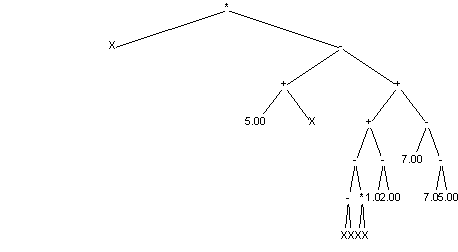The result of each program's run is some value. To find it we have to search the tree backwards (before executing operation in particular node we have to compute expressions held in its children first. Value returned by above tree is described by formula:
`(X*((5.00+X)-((((X-X)-(X*X))+(1.00-2.00))+(7.00-(7.00-5.00)))))`

Elements of such tree may be:

• As nodes:
• arithmetic operators (+,-,/,...)
• logic operators (&&,||,!,...)
• bit operators (&,|,~,...)
• comparisons (>,<,==,...)
• functions (trigonometric, exponential,...)
• condition instruction IF (if condition is fulfilled (first argument), second subtree of second argument is run, if not third)
• iteration – FOR (first argument gives how many times second subtree of second argument have to be run)
• As leaves
• constant values, float and constant point (ie.: 5, 10.5, -11)
• constant values, float and constant point (ex.: X)
• functions calls(ie.: ReadPressedKeyFromKeyboard(), or random())
• functions calls(ex.: printf(), clrscr()) – in case of procedures a value returned by them is a problem (each tree element have to return something to its parent) most often it is accepted that procedures return zero.

Initial population is fulfilled by completely randomly generated programs. During drawing of individuals it is good idea to take care that individuals were not too expanded. We can do that by setting maximum depth or number of nodes in created tree.

crossing over

crossing over basis on exchanging randomly chosen subtrees between individuals. Below example of crossing over is shown (exchanging subtrees are marked with frame):
Situation before crossing over:
First parent: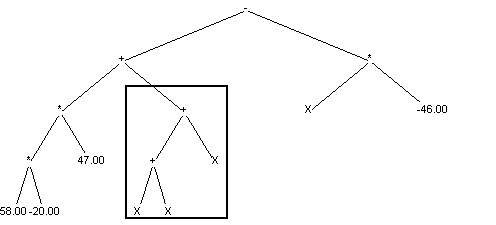Second parent: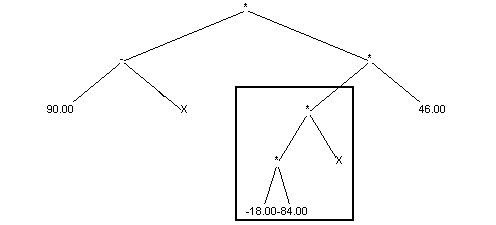Situation after crossing over:
First child: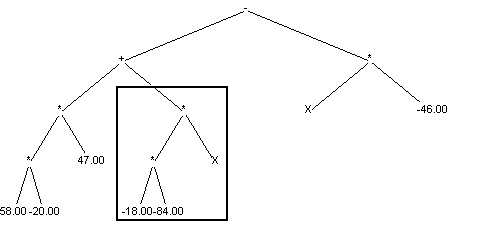Second child: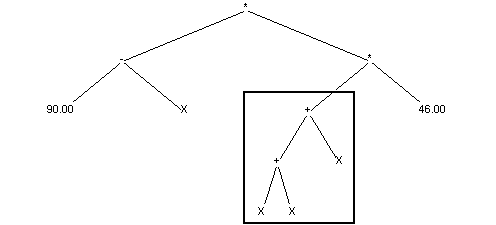Mutation

and generating a new one on its place. Below is an example of such mutation (removed and added subtree is framed)
Individual before mutation: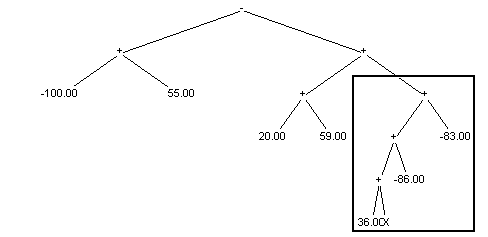Individual after mutation: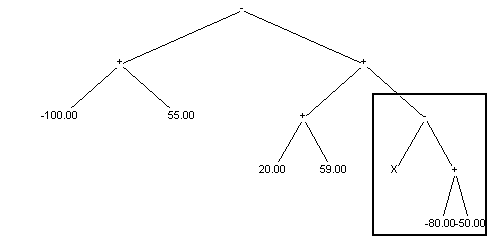Other idea of mutation is an operand change in any tree node. We have to notice here that if new operand have fewer arguments than original (max(a,b)->sin(a)) we have to remove redundant subtrees. If new operator have more arguments (max(a,b)->if(a,b,c))we have to generate missing subtrees.
We can also mutate leaves in the tree. It is the simplest kind of mutation (we don't have to add new nor remove old subtrees), nevertheless causes the least modification if program run which mutated individual represents. This way of mutation is useful while solution (an individual, a program) works almost correctly and only a little gain of coefficients is required (i.e. change of iterations number, treshold of comparisment for instruction IF, etc.).

Estimation function

The main task of estimate function is to check whether given individual performs program we want. Depending on how much results of the program resemble this what we want individual gets proper amount of points. I.e. for solving function approximation task estimate function checks how much does generated function deviates from basis points.

Selection

This part of algorithm is exactly the same as in case of other genetic algorithms usage. As well as roulette wheel also tournament method basis on values of estimation function (they don't need information about individuals to work properly).

Iterative run of all GA steps leads to obtaining the program (at least in approximation) solving given problem. Unfortunately (luckily?) program generated by genetic programming is distant from optimal (as long as now programmers won't loose their jobs). Advantage of genetic programming is a fact that programming in this way doesn't require any work from programmer.

 Web browser program: Artur Mostowski Windows program: Pawel Kopczynski Mentor: Maciej Komosinski Text translation: Piotr Ceglowski